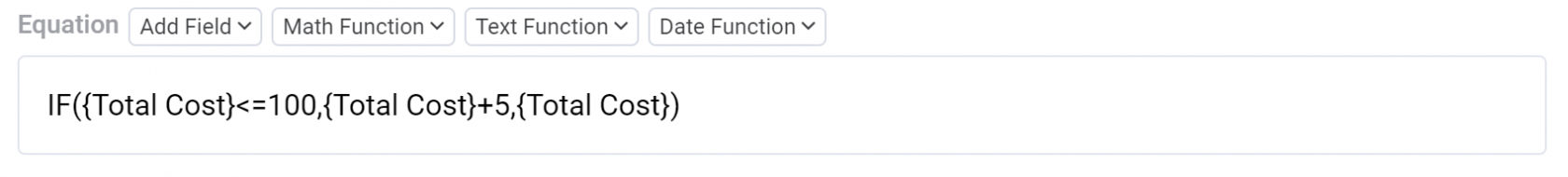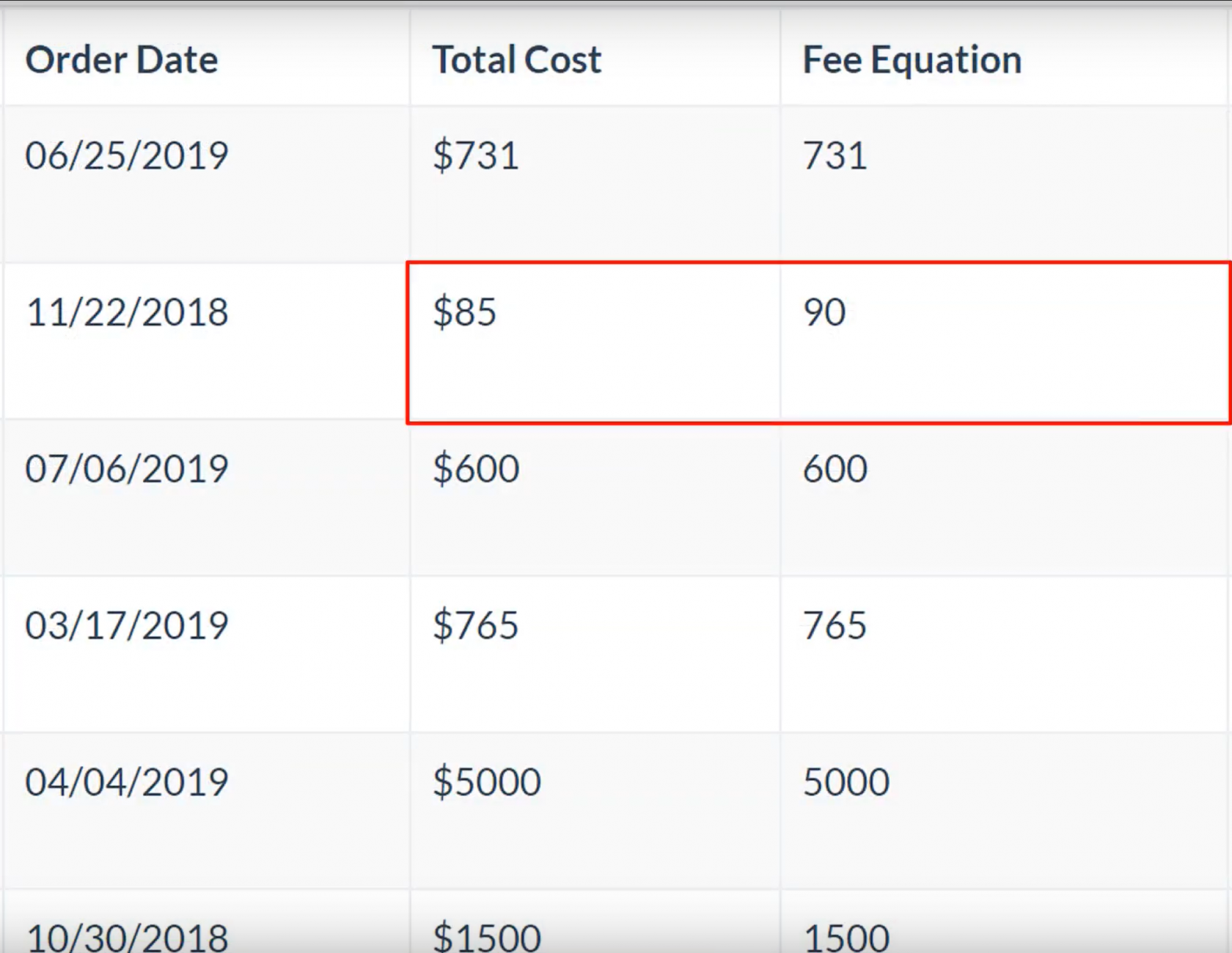The IF function is used within conditional Equation fields to generate a result based on a given condition. IF Statements refer to the IF(formula) statement entered within the equation editor.

IF Statements expect three arguments as input, with each argument separated by a comma. As illustrated in the image below, the first argument is the condition, the second is the desired output if the condition is true, and the third is the desired output if the condition is false. Every IF statement should follow the same formatting illustrated in the image below.For instance, say you have an Orders data table with a Total Cost field, and you would like to create a new field with an updated total cost that adds a \$5 shipping fee for every order with a total cost of less than or equal to \$100. Meaning IF the total cost of the order is under or equal to \$100, add a \$5 shipping fee; otherwise, keep the total cost as-is. You can accomplish this by creating a new Equation field and, within the Equation Editor, type "IF" followed by a left parenthesis. Then enter the three arguments of (condition, true, false) as illustrated below:Let's breakdown the 3 arguments of this IF Statement with the following illustration:The following image displays the results of this conditional IF equation. As you can see, only Total Cost values under or equal to \$100 have been calculated to a new value of plus +\$5. Total Cost values above \$100 remain the same.